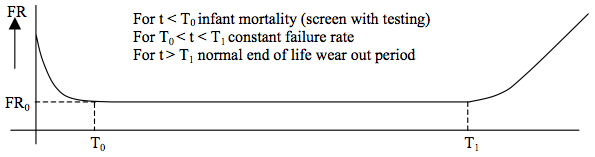ContactNorth America 732 542-7880
Europe 353(91)552432

# Explanation of Life Projection and F.I.T. Calculations

Home > Tech Tools > Technical Papers > Explanation of Life Projection and F.I.T. Calculations

## LIFE PROJECTION

The calculations for the life projections supplied are based on the work of John D. Moynihan as described in “Selection and Application of Capacitors”, Second Edition, Copyright 1987 by Components Technology Institute Inc.

There are two equations that apply to life projection. The first is the Voltage Power Law (for constant temperature) and the second is the Temperature Rule (for constant voltage). These principles are described below and the single equation derivation follows.

Voltage Power Law

L1/L2 = (E2/E1)n
Where:
L1 = Test time in hours
L2 = Projected life expectancy in hours
E1 = Test voltage applied
E2 = Voltage at which the life is projected
n = Exponent as a function of the voltage stress ratio

The exponent n comes from empirically determined values, where n = 2 (25% to 50% rated voltage), n = 3 (50% to 100% rated voltage) and n = 5 (100% to 140% rated voltage). This describes a step function, which is plotted and a line drawn from the (n, %Vr) points (2, 25%) to (5, 140%). An equation can be determined by the slope of this line and is derived as n = 2.6087[(E2/E1) + 0.5167].

Temperature Rule

L1/L2 = 2[(T1 – T2)/n]
Where:
L1 = Test time in hours
L2 = Projected life expectancy in hours
T1 = Test temperature applied
T2 = Temperature at which the life is projected
n = 0C rule for the temperature stress (n = 10 for organic materials)

This rule predicts for organic polymers that every 100C increase in application temperature over test temperature results in 1⁄2 the life, and conversely every 100C decrease in temperature below the test temperature results in 2x the life. Electronic Concepts tests polymers at the maximum rated operating temperature.

Therefore the Temperature Rule equation is reduced to a factor resulting in a multiplier relative to each 100C decrease in temperature from the test condition temperature. The solution of the Voltage Power Law to yield a life projection based on the voltage at constant temperature is then subjected to a multiplier as determined by:

2[(T1 – T2)/10]

Combining the two expressions above for one uniform equation yields:

Projected life = L2 = {L1/ [(E2/E1){2.6087[(E2/E1) + 0.5167]}]}{2[(T1 – T2)/10]}

Ex. 1. – Example of life calculation:
What is the projected life of a metallized polypropylene capacitor at 700C and 550vdc operation based on accumulated test data of 7680 hours testing at 1235vdc and 1050C?

Projected Life
= {7680/[(550/1235){2.6087[(550/1235)+0.5167]}]}{2[(105-70)/10]}
= (7680/0.13132)[(2)(3.5)]
= (58482.25)(11.3137)
= 661,651 hours

Ex. 2. – Adjustment of life for extenuating circumstances
Based on the calculations given above in example 1, provide for the instance in which the capacitor will have to operate for 2,000 hours over the life under a hot start condition of 900C and 1080vdc as an adjustment to the life projection. Also consider that during this hot start condition the ripple current is increased producing an additional 80C internal rise.

For this we consider the temperature to be a total of the ambient and the internal rise or 980C

Projected Life
= {7680/[(1080/1235){2.6087[(1080/1235)+0.5167]}]}{2[(105-98)/10]}
= (7680/0.614644)[(2)(0.7)]
= (12495.03)(1.6245)
= 20,298 hours

Therefore the life expectancy under continuous operation at the hot start condition would be 20,298 hours. The parameters specify that the capacitor will have a maximum usage of 2,000 hours at this condition which accounts for 2,000/20,298 = 0.0985, or approximately 10% of the useful life of the capacitor. Thus returning to the original life projection under normal operating conditions we expect this total life to be decreased by 10%:

Adjusted life = (661,651)(90%) = 595,485.9 hours

## F.I.T. Rate analysis (long term Failures In Time)

The failure rate of capacitors, when plotted against time, takes on the characteristic life curve as shown:FR0 describes the constant failure rate that can be assumed through the normal service period. The constant failure rate is determined from the accumulation of test hours as required in MIL-STD- 690C failure rate “P” at 90% confidence level.

Frtest = (n/N)(1/t) expressed in fit, where 1 fit = 1 failure in 109 component hours.
n = number of failures
N = number of components tested
t = duration of testing in hours

Metallized Polypropylene FIT

As of April 2, 2004 Electronic Concepts, Inc. has tested 11,094 metallized polypropylene capacitors for 10,000 hours each equivalent life with a total of 10 failures due to open or short condition.

The testing was performed at 105C (maximum operating temperature) at 130% to 150% of rated DC voltage.

Frtest = (n/N)(1/t) = (10/11,094)(1/10,000) = 90 x 10-9 = 90 fit

Polypropylene and Foil FIT

As of April 2, 2004 Electronic Concepts, Inc. has tested 6,042 polypropylene and foil capacitors for 10,000 hours each equivalent life with a total of 15 failures due to open or short condition.

The testing was performed at 105C (maximum operating temperature) at 130% to 150% of rated DC voltage.

Frtest = (n/N)(1/t) = (15/6042)(1/10,000) = 248 x 10-9 = 248 fit

J. Bond
Original – June 29, 2004 – first public release
Rev A – July 27, 2004 – Correct typing errors
Rev B – August 11, 2004 – Add prop & foil data to FIT